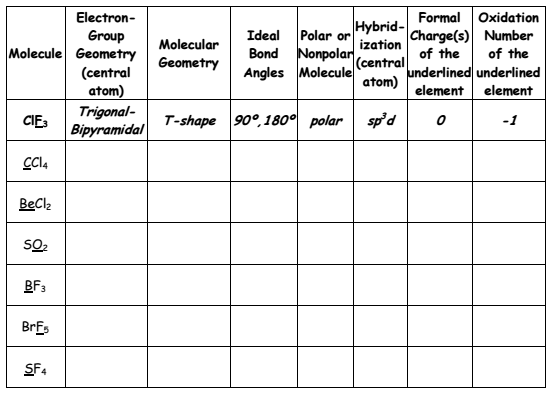# Problem: Fill in the following table (see the ClF3 example). For the determination of formal charge(s) consider the Lewis formula with the smallest formal charges (lowest energy).

###### FREE Expert Solution
85% (323 ratings)
###### Problem Details

Fill in the following table (see the ClF3 example). For the determination of formal charge(s) consider the Lewis formula with the smallest formal charges (lowest energy).What scientific concept do you need to know in order to solve this problem?

Our tutors have indicated that to solve this problem you will need to apply the Hybridization concept. You can view video lessons to learn Hybridization. Or if you need more Hybridization practice, you can also practice Hybridization practice problems.

What is the difficulty of this problem?

Our tutors rated the difficulty ofFill in the following table (see the ClF3 example). For the ...as high difficulty.

How long does this problem take to solve?

Our expert Chemistry tutor, Dasha took 17 minutes and 35 seconds to solve this problem. You can follow their steps in the video explanation above.

What professor is this problem relevant for?

Based on our data, we think this problem is relevant for Professor Rinzler & McGill's class at UF.